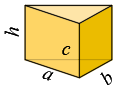### Volume and Surface Area of Triangular PrismDimensions of base

a = , b = , c =

Height

h =

Base perimeter

P = a + b + c

Semiperimeter

p = 0.5·P

Area of base (Heron equation)

A =  p·(pa)·(pb)·(pc)

Volume

V = A·h

Surface area

S = 2·A + h·P

The sum of each two sides must be greater than the third.